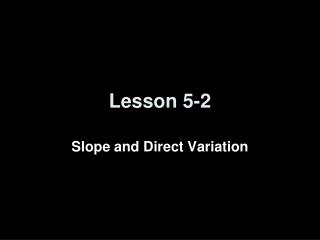# Lesson 5-2 - PowerPoint PPT PresentationDownload PresentationLesson 5-2

Lesson 5-2Download Presentation## Lesson 5-2

- - - - - - - - - - - - - - - - - - - - - - - - - - - E N D - - - - - - - - - - - - - - - - - - - - - - - - - - -
##### Presentation Transcript

1. Lesson 5-2 Slope and Direct Variation

2. Transparency 2 Click the mouse button or press the Space Bar to display the answers.

3. Transparency 2a

4. Objectives • Write and graph direct variation equations • Solve problems involving direct variation

5. Vocabulary • Constant of variation – the number k in equations the form y = kx • Graph – is a plot of an ordered pair (x,y) on the coordinate plane • Family of graphs – graphs and equations of graphs that have at least one characteristic in common • Parent graph – the simplest of the graphs in a family of graphs

6. y y x x x-y Coordinate Plane Point Plotting Slope, m (∆y / ∆x) 5 m = ------ -3 up 7 left 4 right 5 ∆y = 15 rise down 8 run ∆x = -9 (x, y) (-4, 7) (5, -8) rise: ∆y – change in y (7 - -8 = 15) run: ∆x – change in x (-4 – 5 = -9) (x, y) (-4, 7) (5, -8) x – left or right y – up or down

7. Slope formula Simplify. Example 1a Name the constant ofvariation for the equation.Then find the slope of theline that passes through thepair of points. Answer: The constant of variation is 2.The slope is 2.

8. Slope formula Simplify. Example 1b Name the constant ofvariation for the equation.Then find the slope of theline that passes through thepair of points. Answer: The constant of variation is –4.The slope is –4.

9. Example 2 Step 1Write the slope as a ratio. Step 2Graph (0, 0). Step 3From the point (0, 0), move up 1 unit and right 1 unit. Draw a dot. Step 4Draw a line containing the points.

10. Example 3 Step 1Write the slope as a ratio. Step 2Graph (0, 0). Step 3From the point (0, 0), move down 3 units andright 2 units. Draw a dot. Step 4Draw a line containing the points.

11. Simplify. Answer: Therefore, Direct variation formula Replace y with 9 and x with –3. Divide each side by –3. Example 4 • Suppose y varies directly as x, and y = 9 when x = -3 • Write a direct variation equation that relates x and y. Find the value of k.

12. Variables 330 mi r 5.5h Equation Example 5 Travel The Ramirez family is driving cross-country on vacation. They drive 330 miles in 5.5 hours. Write a direct variation equation to find the distance driven for any number of hours. Words The distance traveled is 330 miles, and the time is 5.5 hours. Distance equals rate times time.

13. Original equation Divide each side by 5.5. Simplify. Answer: Therefore, the direct variation equation is Example 5 cont Solve for the rate.

14. Summary & Homework • Summary: • A direct variation is described by an equation of the form y = kx, where k ≠ 0 • In y = kx, k is the constant of variation. It is also the slope of the related graph • Homework: • Pg 268 16-46 even